Editorial Feature

# How Do Mirrors Work?

As we look around a room, we see most objects by the light that is diffusely reflected from them. Diffuse reflection of light takes place when the surface of the object is not smooth as the uneven regions on the surface means the reflected rays leave the surface in many different directions.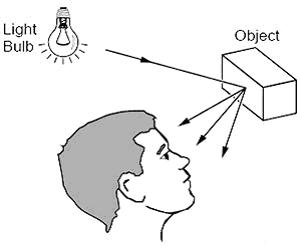Figure 1. Light reflected from objects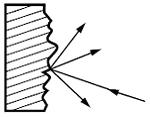Figure 2. Diffuse reflection

## Reflection From Smooth Surfaces

When the surface is smooth, such as in glass or mirrors, the ways in which reflected rays always obey the law of reflection can be demonstrated as below.

## Law of Reflection

The angle of incidence is equal to the angle of reflection.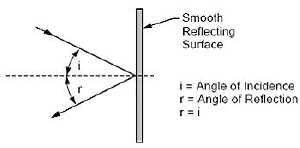Figure 3. Law of reflection

## The Image Formed by Reflection in a Flat Mirror

We see an object because it either has many rays of light being reflected from it or because it is a light source such as a light bulb, the Sun, a star, etc. Each point on that object is a source of light rays.

In the illustration below, the end of the arrow is used as an example of a point on the object from which rays of light would be coming. As the rays from the object are reflected by the mirror, the reflected rays appear to come from the image located behind the mirror at a distance equal to the object's distance from the mirror. The image is called a virtual image since the rays do not actually pass through or come from the image; they just appear to come from the image as illustrated below.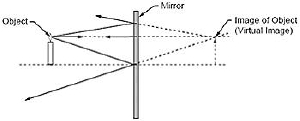Figure 4. Image formed by reflection in a flat mirror

## The Image Formed by a Concave Mirror

A concave mirror is a type of spherical mirror, where the reflective surface protrudes inwards. The focal length is approximately half the radius of curvature. Different points on the curved surfaces of a concave mirror will reflect the light differently. A ray that is both parallel and very close to the optical axis will be reflected by the mirror so that it will cross the optical axis at the “paraxial focal point.”

The paraxial focal point is located a distance of one-half the radius of curvature from the point on the mirror where the optical axis intersects the mirror. The word “paraxial” comes from the Greek “para” or “par” meaning “at the side of, or beside, and axial.” Thus paraxial means beside the axis.

Another ray that is parallel to the optical axis, but not close to the axis, will be reflected by the mirror so that it crosses the optical axis, not at the paraxial focus, but a small distance closer to the mirror. This difference in the axis cross-over points is called spherical aberration.

If the mirror has a cross section that is a parabola instead of a circle, all of the rays that are parallel to the optical axis will cross at the same point. Thus, a parabolic mirror does not produce spherical aberration. This is why the astronomical telescope known as the Newtonian (invented by Isaac Newton) uses a parabolic primary mirror.

For purposes of simplicity, we can make the approximation that spherical mirrors behave almost like parabolic mirrors and determine that the focal length of a spherical mirror is about one-half the radius of curvature of the mirror.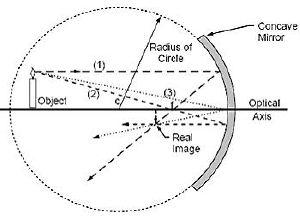Figure 5. Image formed by a concave mirror

In the case where the object is located between the focal point and the mirror, where the object distance is less than the focal length of the mirror, a virtual, upright and enlarged image is obtained. This is the case when looking at yourself in a concave “make-up” mirror, which is described below.

A ray (1) appearing to come from the focal point strikes the mirror and is reflected parallel to the optical axis. A ray (2) parallel to the optical axis is reflected by the mirror so that it goes through the focal point. A ray (3) striking the mirror at the optical axis is reflected so that the angle of reflection is equal to the angle of incidence.

The ray diagram below uses three reflected rays to illustrate how the image can appear to be enlarged and upright. The image formed is a virtual image.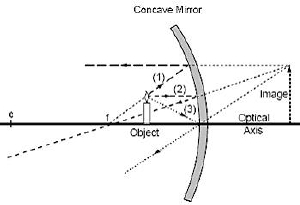Figure 6. Ray diagram with a convex mirror

## The Image Formed by a Convex Mirror

The image formed by a convex mirror is virtual, upright, and smaller than the object. This is illustrated by the ray diagram on the following page. The diagram depicts the three rays that are discussed in the following paragraph. A ray (1) parallel to the optical axis is reflected as if it came from the focal point (f). A ray (2) directed toward the focal point is reflected parallel to the optical axis. A ray (3) striking the mirror at the optical axis is reflected at an angle equal to the angle of incidence.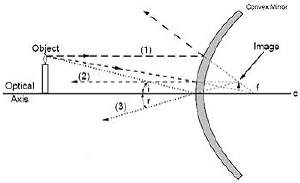Figure 7. Convex mirror ray diagram